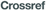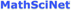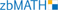0
2013
Impact Factor

# On a Class of Isotopic Connectivity of Gradient-like Maps of the 2-sphere with Saddles of Negative Orientation Type

2019, Vol. 15, no. 2, pp.  199-211

Author(s): Medvedev T. V., Nozdrinova E. V., Pochinka O. V., Shadrina E. V.

We consider the class $G$ of gradient-like orientation-preserving diffeomorphisms of the 2-sphere with saddles of negative orientation type. We show that the for every diffeomorphism $f\in G$ every saddle point is fixed. We show that there are exactly three equivalence classes (up to topological conjugacy) $G=G_1\cup G_2\cup G_3$ where a diffeomorphism $f_1\in G_1$ has exactly one saddle and three nodes (one fixed source and two periodic sinks); a diffeomorphism $f_2\in G_2$ has exactly two saddles and four nodes (two periodic sources and two periodic sinks) and a diffeomorphism $f_3\in G_3$ is topologically conjugate to a diffeomorphism $f_1^{-1}$. The main result is the proof that every diffeomorphism $f\in G$ can be connected to the source-sink'' diffeomorphism by a stable arc and this arc contains at most finitely many points of period-doubling bifurcations.
Keywords: sink-source map, stable arc
Citation: Medvedev T. V., Nozdrinova E. V., Pochinka O. V., Shadrina E. V., On a Class of Isotopic Connectivity of Gradient-like Maps of the 2-sphere with Saddles of Negative Orientation Type, Rus. J. Nonlin. Dyn., 2019, Vol. 15, no. 2, pp.  199-211
DOI:10.20537/nd190209

## References

 Newhouse, S., Palis, J., and Takens, F., “Stable Arcs of Diffeomorphisms”, Bull. Amer. Math. Soc., 82:3 (1976), 499–502Newhouse, S. and Peixoto, M. M., “There Is a Simple Arc Joining Any Two Morse – Smale Flows”, Trois études en dynamique qualitative, Astérisque, 31, Soc. Math. France, Paris, 1976, 15–41Nozdrinova, E. V., “Rotation Number As a Complete Topological Invariant of a Simple Isotopic Class of Rough Transformations of a Circle”, Russian J. Nonlinear Dyn., 14:4 (2018), 543–551Blanchard, P. R., “Invariants of the NPT Isotopy Classes of Morse – Smale Diffeomorphisms of Surfaces”, Duke Math. J., 47:1 (1980), 33–46Grines, V., Medvedev, T., and Pochinka, O., Dynamical Systems on $2$- and $3$-Manifolds, Dev. Math., 46, Springer, New York, 2016, XXVI, 295 pp.von Kerékjártó, B., “Über die periodischen Transformationen der Kreisscheibe und der Kugelflache”, Math. Ann., 80:1 (1919), 36–38Newhouse, S., Palis, J., and Takens, F., “Bifurcations and Stability of Families of Diffeomorphisms”, Inst. Hautes Études Sci. Publ. Math., 1983, no. 57, 5–71Milnor, J., Lectures on the $h$-Cobordism Theorem, Princeton Univ. Press, Princeton, N.J., 1965, v+116 pp.Katok, A. and Hasselblatt, B., Introduction to the Modern Theory of Dynamical Systems, Encyclopedia Math. Appl., 54, Cambridge Univ. Press, Cambridge, 1995, 802 pp.Rolfsen, D., Knots and Links, Math. Lect. Ser., 7, Publish or Perish, Inc., Berkeley, Calif., 1976Banyaga, A., “On the Structure of the Group of Equivariant Diffeomorphisms”, Topology, 16:3 (1977), 279–283